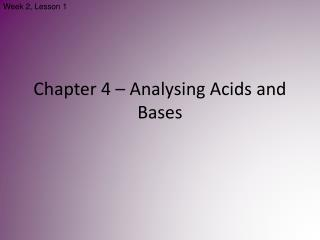DownloadDownload PresentationChapter 4 – Analysing Acids and Bases

# Chapter 4 – Analysing Acids and Bases

Télécharger la présentation## Chapter 4 – Analysing Acids and Bases

- - - - - - - - - - - - - - - - - - - - - - - - - - - E N D - - - - - - - - - - - - - - - - - - - - - - - - - - -
##### Presentation Transcript

1. Week 2, Lesson 1 Chapter 4 – Analysing Acids and Bases

2. Acid Base Chemistry Revisited • Acids are proton donors. • Bases are proton acceptors. • Acid-base reactions involve the transfer of a proton from an acid to a base.

3. Strong and Weak • Strong Acid: ionises almost completely in water. • Weak Acid: does not ionise completely in water. • Strong Base: readily accept protons. • Weak Base: accepts protons only to limited extent.

4. pH • The concentration of H3O+ ions in a solution is referred to as the solution’s acidity. • Acidity is measured using a logarithmic scale, called the pH scale. • pH = -log[H3O+]

5. Indicators • An indicator is used during acid-base titration to identify the equivalence point of the reaction. • An acid-base indicator is a substance whose colour depends on the concentration of H3O+ ions in solution. • Indicators are acids with their acid form being one colour and the conjugate base being another.

6. Indicators & their Range • The indicator must be chosen carefully to ensure that the point during the titration where the indicator changes colour, the end point, closely matches the equivalence point of the reaction. • If we look at the pH changes that take place between a strong acid and a strong base, we would see a graph similar to 4.4a (page 40). At the end point, addition of a very small volume of strong acid produces a large change in pH. This is referred to as the sharp end point. • In the case between a strong acid and base, the equivalence point falls close to 7.0

7. Indicators & their Range cont… • When a weak acid is titrated with a strong base, or vice versa, there is a more gradual change in pH around the end point. • In this situation, only indicators whose colour changes within the end point range can be used. • When a strong acid reacts with a weak base, the equivalence point occurs between pH 3 and 7. • Methyl orange changes colour over this range and as such would be a suitable indicator. • The equivalence point for a reaction between a weak acid and a strong base occurs between pH 7 and 11. • A suitable indicator for this would be phenolphthalein.

8. Week 2, Lesson 2 Back Titration • Some acids and bases are so weak that they do not produce a sharp colour change at the end point of a titration. • A technique called back titration is used to overcome this problem. • The procedure of a back titration has two parts: • If the substance to be analysed is a weak acid, it is mixed with an excess of strong base. The original amount of the base is known. All of the weak acid reacts in stoichiometric proportions, leaving some of the strong base unused. • The unused strong base is titrated as normal with a standard solution of a strong acid. Knowing the original amount of strong base and the amount of strong base left unused, it is possible to work bask to find the amount of weak acid present. The same procedure can be used to determine the concentration of a weak base by adding an excess of a strong acid.

9. Back Titration cont… Determining the Concentration of Ammonium Ion in Lawn Fertiliser • Nitrogen-containing ions, such as ammonium ions, are required by plants to synthesise materials needed for healthy growth. • For this reason, lawn fertilisers often contain a high proportion of ammonium sulfate or ammonium nitrate. • The ammonium ion (NH4+) acts as a very weak acid, and a direct titration with a base does not give a sharp end point.

10. Determining Concentration cont… • A measured mass of fertiliser is added to a solution containing an excess amount of sodium hydroxide. The ammonium ions react with hydroxide ions: NH4+(aq) + OH-(aq)  NH3(aq) + H2O(l) The mixture is boiled to ensure complete reaction of the ammonium ions. Some of the the hydroxide ions are consumed by the reaction but others remain unreacted. 2. The quantity of excess hydroxide ions is found by titrating the solution with a standard solution of hydrochloric acid: HCl(aq) + NaOH(aq)  NaCl(aq) + H2O(l) Knowing the original quantity of sodium hydroxide and the amount in excess after reaction with the fertiliser, we can calculate, by subtraction, the quantity of hydroxide ions that reacted with the fertiliser. The concentration of ammonium ions in the fertiliser can then be calculated by working backwards.

11. Back Titration Example… A 1.50g sample of lawn fertiliser was boiled with 25.00mL of 0.9987M sodium hydroxide solution. When no further ammonia gas was evolved from the mixture, it as cooled and titrated with 0.2132M hydrochloric acid, using phenolphthalein as an indicator. A titre of 19.78mL was required. Calculate the percentage of ammonium ions in the fertiliser. STEP 1: Find the original amount of NaOH used. STEP 2: Find the amount of NaOH that did not react with NH4+ ions. STEP 3: Find the amount of NaOH that reacted with NH4+ ions. STEP 4: Find the amount of NH4+ in the fertiliser sample. STEP 5: Find the percentage of NH4+ in the sample.